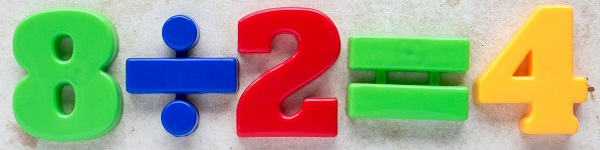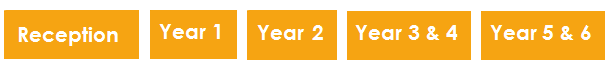School year > Subject guides > Maths in Year 1

# Maths in Year 1 (age 5–6)At this age, here are some things your child is likely to be doing:

• Reading and writing numerals to at least 100 in numerals and in words
• Finding one more or less than any number
• Read, write and interpret mathematical statements involving addition (+), subtraction (-) and equals (=)
• Recognising, finding, naming and writing simple fractions of a length, shape, set of objects or quantity
• Measure and record the length, height, weight or volume of different objects
• Recognise and talk about the value of different denominations of coins and notes
• Tell the time to the hour and half past the hour, drawing the hands on a clock face to show these times
• Ordering and arranging objects in patterns and sequences
• Recognise and name common 2D and 3D shapes including squares, circles and pyramids
• Describing position, direction and movement

### Different ways to teach and learn maths at school

Maths will normally be taught on a daily basis, in a timetabled lesson, but children practise and use their maths throughout the school day.

From the outset, there will be a lot of focus on developing basic maths skills, such as remembering basic addition and subtraction facts, for example, 9 – 6 and 4 + 6. Teachers often set aside part of each lesson to devote to this learning.

### Assessment

Assessment for young children is informal and discrete and helps to inform the teacher’s planning so they can support each child in their class.